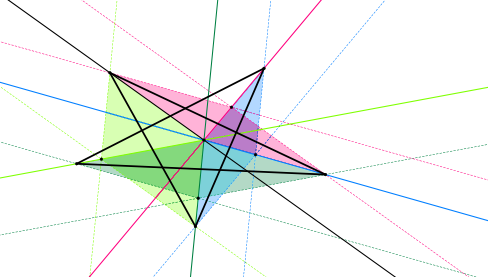# PentagonsA while back I developed a mild obsession with pentagons (mathematical ones, not symbolistic!) It started when I discovered some beautiful (simple and to me, unknown) theorems of quadrangles, such as  Varignon’s theorem. I already came across Miquel’s pentagon theorem, and wondered what other gems I could find.

Here is what I found: Pentagons (2.4 MB PDF).

My search was on the surface a bit disappointing: pentagons as such are not widely studied. I guess it is because some more general theorems (that apply to general polygons) contain the theory, and the specifics as applied to pentagons are not so interesting.

Nevertheless, I did discover a few theorems, and the journey took me into some very interesting corners of geometry; a very rewarding experience. I started to collect these into a document, which is shared below. It’s not comprehensive or complete; there are a few gaps.

(At some stage I may return to look at this again. In particular, there are many theorems of the type “if there is n, there is n+1”, which seems to me to hint at a very general theorem which can be used to prove a bunch of specifics.)

Also, when I started, I did not realise how many of the theorems will generalise to general polygons, so the collection looks a bit silly in retrospect (kind of like listing all the properties of the number 8 that equally apply to even numbers).

Even so, what’s done is done, and can perhaps satisfy someone else’s curiosity.

Here is the list of contents:

1. Notation
1. Standard labeling
2. Cycle notation (I introduce a convention that to me makes it easier to keep track of symbols in my head.)
3. Area
2. Five points in a plane
3. General Pentagons
1. Monge, Gauss, Ptolemy (Theorems that have analogues for quadrangles. For example, one theorem relates the areas of six triangles spanned by certain vertices of the pentagon.)
2. Cyclic Ratio Products (alla Ceva en Melenaus) (Theorems involving products of cevians and other ratios.)
3. Miquel (Thereoms analoguous to Miquel’s theorem for triangles.)
4. Conics (Circumscribed and inscribed conics are uniquely determined by a pentagon.)
5. Complete Pentagons (Includes Miquel’s pentagram theorem.)
6. The Centroid Theorem (A general theorem about centroid applied to pentagons.)
4. Special Pentagons (Including how to construct these pentagons.)
1. Cyclic Pentagons (Pentagons with vertices on a circle.)
2. Tangent Pentagons (Pentagons with all sides tangent to a circle.)
3. Orthocentric Pentagons (Pentagons whose altitudes intersect in a single point.)
4. Mediocentric Pentagons (Pentagons whose medians intersect in a single point.)
5. Paradiagonal Pentagons (Pentagons whose diagonals are parallel to oposite sides. Also called golden pentagons, affine regular pentagons, and equal area pentagons.)
6. Equilateral Pentagons (Pentagons with all sides of equal length.)
7. Equiangular Pentagons (Pentagons with all interior angles equal.)
8. Brocard Pentagons (Pentagons that have a Brocard point.)
9. Classification By Subangles (What the relationships between subangles imply for the pentagon.)